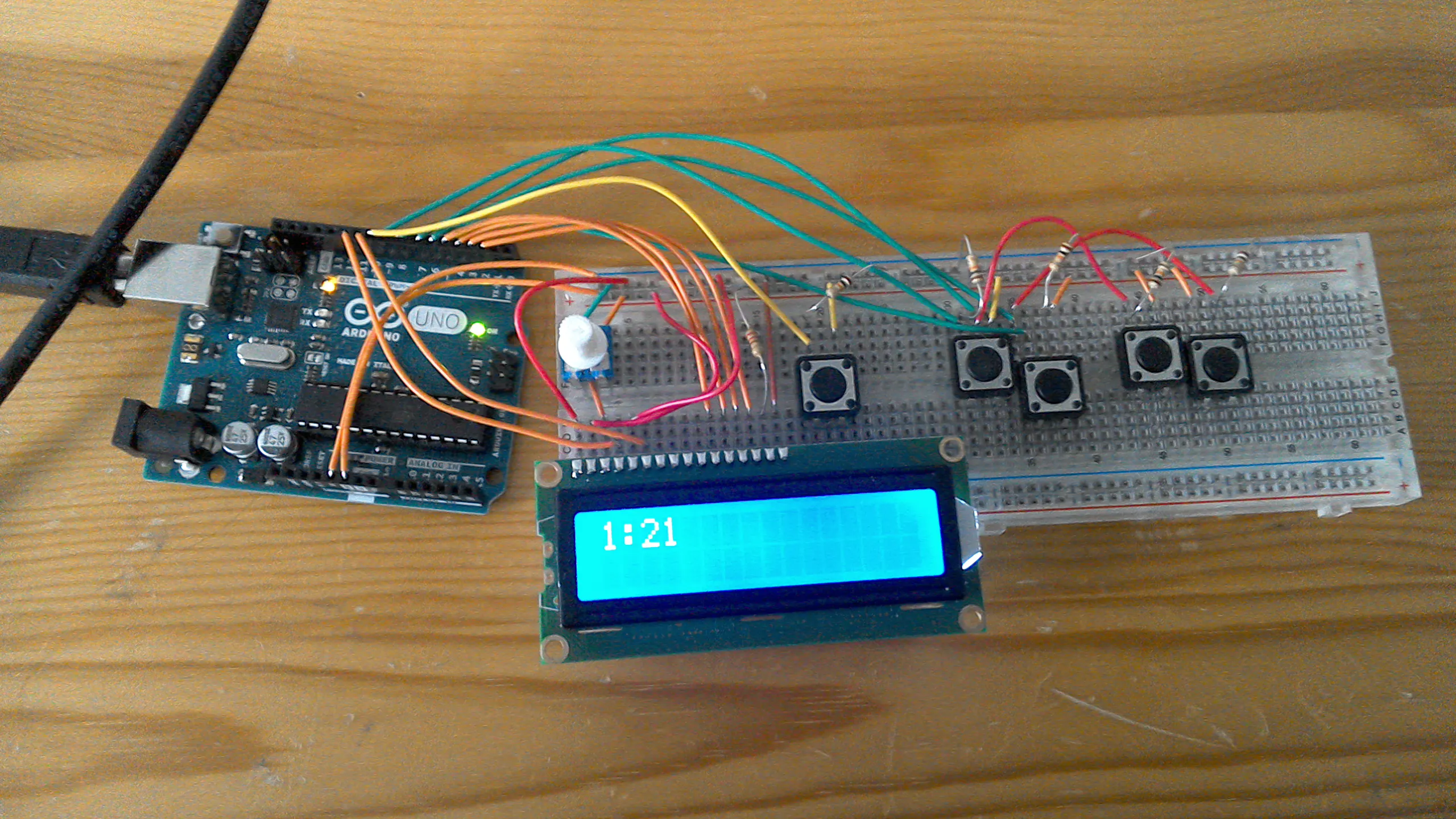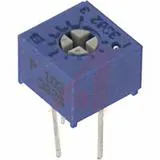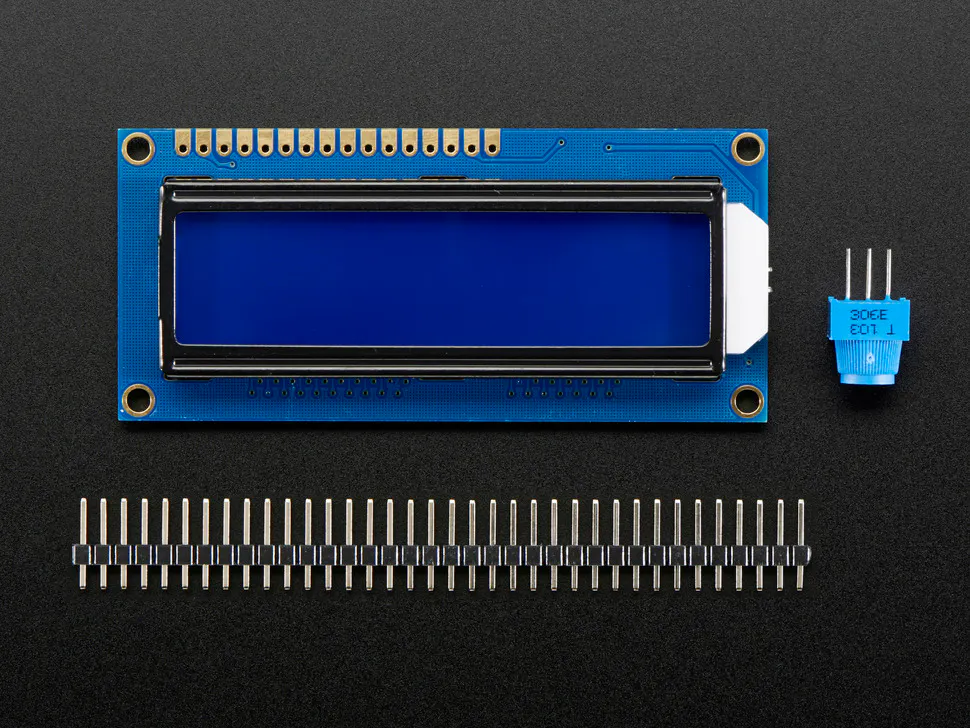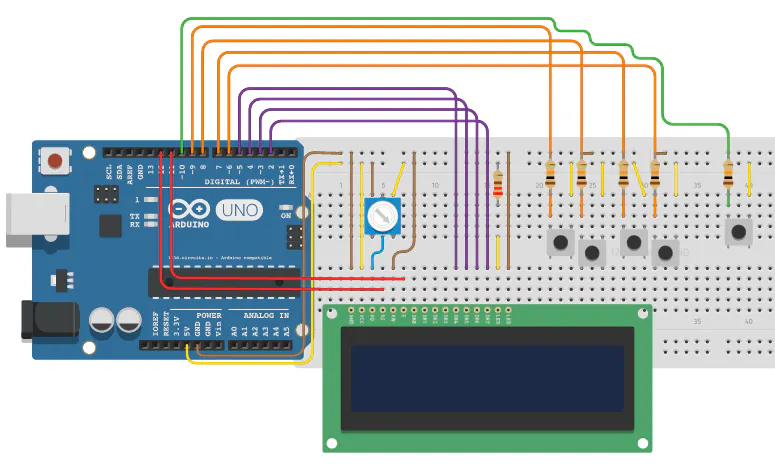# Digital Clock

Basically a digital clock that can have the time changed at the press of a button.

IntermediateWork in progress16,363## Things used in this project

### Hardware componentsArduino UNO
×1Single Turn Potentiometer- 10k ohms
×1Adafruit Standard LCD - 16x2 White on Blue
×1SparkFun Pushbutton switch 12mm
×1
 220 ohm resisitors
×1Resistor 10k ohm
×5

### Software apps and online servicesArduino IDE

## Schematics

### Schematic of wiring.

Just the schematic.## Code

### My code!

C/C++
Here is the code in case anybody wants to try it for themselves.
```#include <LiquidCrystal.h>
//to change time, hold chanage time button for 1 second, then use hour chnage and minute change buttons.

LiquidCrystal lcd(12, 11, 5, 4, 3, 2);
int hour = 12;
int minutes = 0;
int seconds = 0;
int decBtn = 10;
int hrUp = 9;
int hrDwn = 8;
int minUp = 7;
int minDwn = 6;
int hrUpDec = 0;
int hrDwnDec = 0;
int minUpDec = 0;
int minDwnDec = 0;

void setup() {
lcd.begin(16, 2);
pinMode(decBtn, INPUT);
pinMode(hrUp, INPUT);
pinMode(hrDwn, INPUT);
pinMode(minUp, INPUT);
pinMode(minDwn, INPUT);
}

void loop() {
if (digitalRead(hrUp) == HIGH && hrUpDec == 0) {
hrUpDec = 1;
hour = hour + 1;
} else if (digitalRead(hrUp) == LOW) {
hrUpDec = 0;
}
if (digitalRead(hrDwn) == HIGH && hrDwnDec == 0) {
hrDwnDec = 1;
hour = hour - 1;
} else if (digitalRead(hrDwn) == LOW) {
hrDwnDec = 0;
}
if (digitalRead(minUp) == HIGH && minUpDec == 0) {
minUpDec = 1;
minutes = minutes + 1;
} else if (digitalRead(minUp) == LOW) {
minUpDec = 0;
}
if (digitalRead(minDwn) == HIGH && minDwnDec == 0) {
minDwnDec = 1;
minutes = minutes - 1;
} else if (digitalRead(minDwn) == LOW) {
minDwnDec = 0;
}
seconds = 0;
lcd.clear();
if (minutes == 60) {
minutes = 0;
hour = hour + 1;
}
if (minutes < 0) {
minutes = 59;
}
if (hour == 13) {
hour = 1;
}
if (hour < 1) {
hour = 12;
}
lcd.print(hour);
lcd.print(":");
if (minutes < 10) {
lcd.print(0);
}
lcd.print(minutes);
delay(20);
} else if (digitalRead(decBtn) == LOW) {
lcd.clear();
if (seconds == 60) {
seconds = 0;
minutes = minutes + 1;
}
if (minutes == 60) {
minutes = 0;
hour = hour + 1;
}
if (minutes < 0) {
minutes = 59;
}
if (hour == 13) {
hour = 1;
}
if (hour < 1) {
hour = 12;
}
lcd.print(hour);
lcd.print(":");
if (minutes < 10) {
lcd.print(0);
}
lcd.print(minutes);
delay(1000);
lcd.clear();
seconds = seconds + 1;
}
}
```

## Credits

### SharKode

1 project • 6 followers